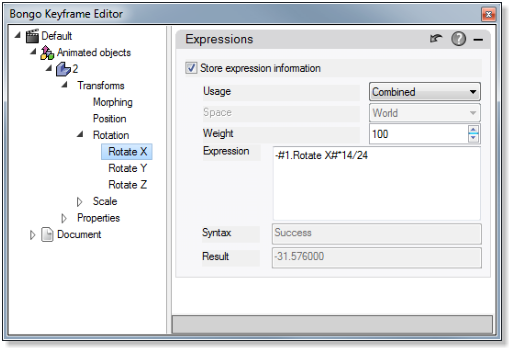## What are expressions?

An expression describes how a set of values defines a new value. It consists of numbers, parameters, operators and functions. Operators and functions define how numbers together with parameters evaluate to a numeric result. Rules specify which symbols together form a valid expression.

### Expressions in Bongo

Expressions in Bongo support basic operations: addition [+], subtraction [-], multiplication [] and division [/]. They are operators as they combine two values into one. Fifth supported operator is power [^]. Valid expressions constructed of numbers and operators are for example 54+7 and 7^2+5^2.

Animated objects’ transform parameters and properties can be used as components in an Expression. The name of the object and the property or transform parameter, separated by a dot, is typed inside number signs. For example the value for scaling along X-axis for an animated object named CoffeeCup has the form #CoffeeCup.Scale X#.

Functions can be applied. They take in an argument and return a value.

Supported mathematical functions are square root [sqrt], floor [floor], ceiling [ceil], absolute value [abs] and natural logarithm [log]. Supported trigonometric functions are sine [sin], cosine [cos], tangent [tan], arcsine [asin], arccosine [acos], arctangent [atan].

The function’s argument must always be in parentheses. The argument can be any objects’ transform parameters or properties (as described above) and/or numbers and mathematical operations: e.g.

sin(#Object0.RotateZ#*0.01745)

sqrt(3+#Hinge.WorldAngle#^2) atan(#ObjectA.PositionY#+cos(#Rod.ObjectAngle#*Pi/180)).

The argument of the trigonometric functions is in radians, not in degrees.

Pi [pi] is a constant (3.14159265359…). It requires no parentheses.

Parentheses can be used to change the precedence of operators and functions. For example, multiplication has a higher precedence than addition so 3+2*2 evaluates to 7 but with parentheses around the addition (3+2)*2 it evaluates to 10.

A parameter can have multiple expressions by specifying the tick range they are valid in. The tick range is specified using [Start-Stop]. For example:

[0-50] #CoffeeCup.Scale X# [51-99] #CoffeeCup.Scale Z#

You can also use commas to separate the ticks:

[1,20,25] #CoffeeCup.Scale X# [30,35,40] #CoffeeCup.Scale Z#

The letter t has a special meaning in Expressions. It is a variable worth the current tick. So for example, expression 10*t+4 evaluates to 4 at tick 0 and 994 at tick 99.

Minus sign in front of a number or parameter negates the value. White spaces are allowed around numbers and parameters.

Expressions can be combined or add with keyframe-data using the weight values.

### Examples of expressions

• 3.4 + 3 * sin( 2 * 3.14159265 * #ObjectA.Rotate X# / 360 )
• -abs( t / 10 )
• ( 3 + t ) / (4 + 3 * sin( t ) ) / ( ( cos( t ) + 2 ) * log( #Box.Position X# ) )### Expressions and 3D-tweening

Bongo uses 3-dimensional tweening for position by default. This means that all the 3 coordinates x, y and z aare tweened together. With 3D-tweening disabled the coordinates are treated separately as numbers. Controlling position with expressions or using Position parameters for arguments is only possible when 3-dimensional tweening is disabled.

This can be done in the Bongo Animation Manager by right clicking the object and unchecking 3D Tweening Enabled.